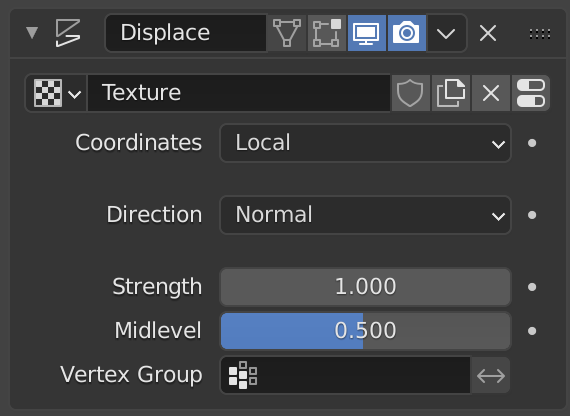# Displace Modifier¶

The Displace modifier displaces vertices in a mesh based on the intensity of a texture. Either procedural or image textures can be used.

The displacement can be along a particular local axis, along the vertex normal, or the separate RGB components of the texture can be used to displace vertices in the local X, Y and Z directions simultaneously (sometimes referred to as Vector Displacement).

## Options¶The Displace modifier.

Texture

The name of the texture from which the displacement for each vertex is derived. If this field is empty, the modifier defaults to 1.0 (white).

Coordinates

The texture coordinate system to use when retrieving values from the texture for each vertex.

See common masking options for a complete reference.

Direction

The direction along which to displace the vertices. Can be one of the following:

X, Y, Z

Displace along an axis.

Normal

Displace along the vertex normal.

Custom Normal

Displace along (averaged) custom normals, instead of vertex normals.

RGB to XYZ

Displace along local XYZ axes individually using the RGB components of the texture (Red values displaced along the X axis, Green along the Y, Blue along the Z).

Space

With a direction set to X, Y, Z, or XYZ the modifier can either displace along local or global axes.

Strength

The strength of the displacement. After offsetting by the Midlevel value, the displacement will be multiplied by the Strength value to give the final vertex offset.

$$vertex_offset = displacement × Strength$$

A negative strength can be used to invert the effect of the modifier.

Midlevel

The texture value which will be treated as no displacement by the modifier. Texture values below this threshold will result in negative displacement along the selected direction, while texture values above it will result in positive displacement.

$$displacement = texture_value - Midlevel$$

Recall that color/luminosity values are typically between (0.0 to 1.0) in Blender, and not between (0 to 255).

Vertex Group

The name of a vertex group which is used to control the influence of the modifier. If left empty, the modifier affects all vertices equally.

Invert <->

Inverts the influence of the selected vertex group, meaning that the group now represents vertices that will not be deformed by the modifier.

The setting reverses the weight values of the group.